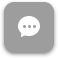Call Now 855-300-1469

# BUS 5602 Essentials of Business Development 2

#### Course Description

Builds on 5601. Examines and uses concepts, methods and models from the functional business areas of statistics, finance, management and law. Requires students to integrate each component into a complete business plan.

#### Course Objectives

The objective of this course is to help business students learn fundamental business concepts and apply them by developing a business plan. This 8 week course is the second in a series of two courses. Students will learn the basics of finance, statistics, management and business law.

### Week 1

##### Lecture: Course Introduction

Outcomes

• Compute the future value of an investment made today
• Compute the present value of cash to be received at some future date
• Compute the return on an investment
• Compute the future value of multiple cash flows
• Compute the present value of multiple cash flows
• Understand how interest rates are quoted
• Understand EAR and APR

### Week 2

##### Lecture: Capital Investment Decisions

Outcomes

• Understand the importance of Capital Budgeting
• Understand the NPV method and its advantages
• Understand the Payback method and its shortcomings
• Understand the IRR method and its strengths & weaknesses
• Understand how to determine the relevant cash flows for a proposed project
• Understand how to analyze a project’s estimated cash flows
• Understand how to evaluate an estimated NPV
• Understand the relationship between Risk and Return
• Learn what Capital Market History tells us about Risk and Return
• Understand the two central lessons of Capital Market History
• Understand an efficient capital market
• Understand systematic and unsystematic risk
• Discuss the benefits of diversification
• Understand the Beta coefficient
• Discuss SML
• Understand CAPM

### Week 3

##### Lecture: Statistics Introduction

Outcomes

• Answer the question “Why should managers study statistics?”
• Calculate each measure of central location
• Understand the strengths and weaknesses of each measure of central location
• Understand and measure range, IOR and standard deviation
• Create histograms, box plots, bar charts, pie charts and line charts
• Interpret a scatter plot, and two statistics: the correlation coefficient and the coefficient of determination (r2)
• Describe the main characteristics of a normal distribution, compute and interpret z-scores and calculate probabilities using the standard normal distribution
• Understand the concept of statistical inference

### Week 4

##### Lecture: Statistical Inference

Outcomes

• Discuss the fundamentals of experimental design and analysis
• List the key ideas associated with statistical hypotheses, how to formulate hypothesis tests and the role of probability in assessing the strength of sample evidence
• Perform hypothesis tests for samples with a single population and more than one population
• Perform hypothesis tests on samples with unrelated or related groups
• Describe the basics behind ANOVA testing and multiple regressions

### Week 5

##### Lecture: Management Introduction

Outcomes

• List the four functions of management and the roles of a manager
• Discuss the causes behind the global shift in the business world
• Describe the factors that a manager must consider before entering the global environment
• Explain the aspects and differences between vertical and horizontal organization structure
• Compare and contrast the three types of departmentalization

### Week 6

##### Lecture: Human Resources

Outcomes

• Describe the HR process
• Understand the benefits of using teams
• Describe the types of teams
• Understand the problems that can arise from using teams and the management strategies that can be used to deal with the problems
• Describe the benefits of MIS

### Week 7

Outcomes

• Describe a property-based legal system
• Discuss the sources of law
• List the sources of values for business ethics
• Summarize the principles of contract law

### Week 8

##### Lecture: Agency Law

Outcomes

• Understand the principles of agency law
• Name the factors you need to consider when selecting a business organizational firm
• Know different types of business organization firms
• Be able to explain the important concepts in the Sherman Act and Clayton Act
• Describe the role of the FTC
• Understand and explain the importance of the Sarbanes-Oxley Act of 2002, the Securities Act of 1933, and the Securities Exchange Act of 1934
• Understand the major items covered by US Employment Law
• Understand the importance of the Wagner Act and the Taft-Hartley Act

The course description, objectives and learning outcomes are subject to change without notice based on enhancements made to the course. May 2011